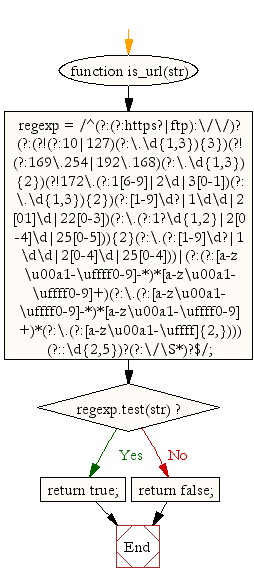# JavaScript: Check whether a given value is an valid url or not

## JavaScript validation with regular expression: Exercise-9 with Solution

Write a JavaScript function to check whether a given value is an valid url or not.

Sample Solution:-

HTML Code:

``````<!DOCTYPE html>
<html>
<meta charset="utf-8">
<title>JavaScript function to check whether a given value is an url or not</title>
<body>

</body>
</html>
```
```

JavaScript Code:

``````function is_url(str)
{
regexp =  /^(?:(?:https?|ftp):\/\/)?(?:(?!(?:10|127)(?:\.\d{1,3}){3})(?!(?:169\.254|192\.168)(?:\.\d{1,3}){2})(?!172\.(?:1[6-9]|2\d|3[0-1])(?:\.\d{1,3}){2})(?:[1-9]\d?|1\d\d|2\d|22[0-3])(?:\.(?:1?\d{1,2}|2[0-4]\d|25[0-5])){2}(?:\.(?:[1-9]\d?|1\d\d|2[0-4]\d|25[0-4]))|(?:(?:[a-z\u00a1-\uffff0-9]-*)*[a-z\u00a1-\uffff0-9]+)(?:\.(?:[a-z\u00a1-\uffff0-9]-*)*[a-z\u00a1-\uffff0-9]+)*(?:\.(?:[a-z\u00a1-\uffff]{2,})))(?::\d{2,5})?(?:\/\S*)?\$/;
if (regexp.test(str))
{
return true;
}
else
{
return false;
}
}

console.log(is_url("http://www.example.com"));
console.log(is_url("https://www.example.com"));
console.log(is_url("www.example.com"));
```
```

Sample Output:

```true
true
true
```

Flowchart:Live Demo:

See the Pen javascript-regexp-exercise-8 by w3resource (@w3resource) on CodePen.

Improve this sample solution and post your code through Disqus

What is the difficulty level of this exercise?

﻿

## JavaScript: Tips of the Day

JavaScript: eval() function

```const sum = eval('10*10+5');
```

eval evaluates codes that's passed as a string. If it's an expression, like in this case, it evaluates the expression. The expression is 10 * 10 + 5. This returns the number 105.

Ref: https://bit.ly/323Y0P6